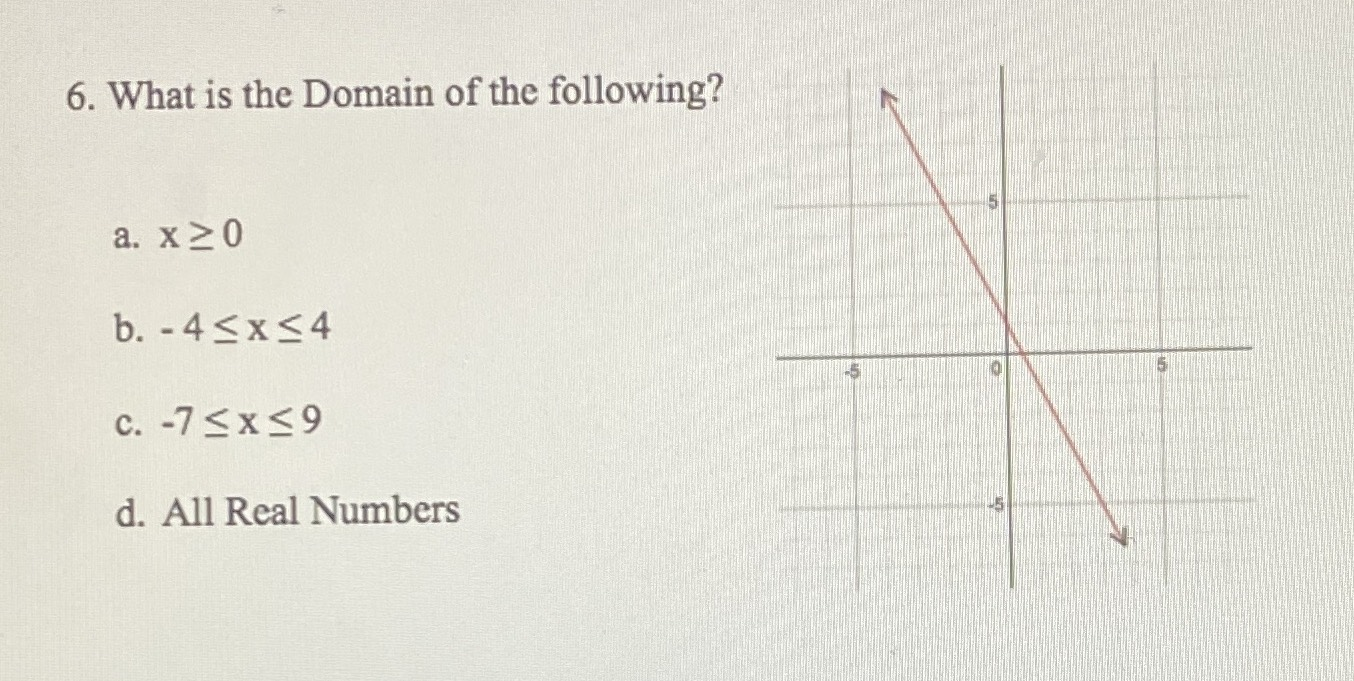### ¿Todavía tienes preguntas de matemáticas?

Pregunte a nuestros tutores expertos
Algebra
Pregunta6. What is the Domain of the following?

a. $$x \geq 0$$

b. $$- 4 \leq x \leq 4$$

c. $$- 7 \leq x \leq 9$$

d. All Real Numbers